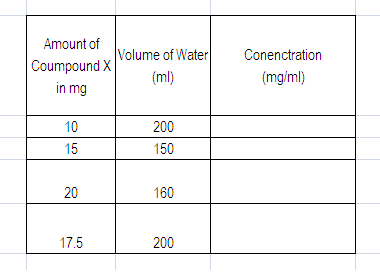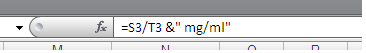# Excel - Add Units to a Function or Formula

In excel it is possible to add specialist units to the end of a calculation, function or formula (eg average and sum) and this can be reported in a single cell. The following method detailed in this tutorial allows the formula to be scrolled, dragged or expanded using the standard excel functions. The following code is the generic method to add units to an function or formula:

=Calculation(Range)&" units"

The units of milligrams per millilitre (mg/ml) could be added to the average (mean) calculation of cell A2 through to A8. The cell contents would be written as:

=AVERAGE(A2:A8)&" mg/ml"

This would appear as 5.5 mg/ml in the cell and as in a single cell is suitable for export into word or other presentation software.

## Worked Example - Excel Add Units to a Formula

In this example the concentration of 4 solutions is required. Excel can tabulate and calculate the values which then can be exported to a separate processing program. Concentration is calculated by dividing the weight, milligrams (mg) in this example, by the volume of solution, millilitres (ml) in this example.

The data for the worked example is tabulated below:To calculate the concentration the following formula is added to the function bar:
For this example the weight is listed in column S while the volume is in column T.This produces a table of results that looks like this:Presently there are no units added to the concentration column, this can be adjusted using the information stated in the introduction to alter the formula in the excel function bar .

The &" xxx" is added after the excel function as show in the image below:This automatically displays the result of the simple formula along with the desired units.This function or formula can the be applied to the entire table using the standard excel operations.

In the worked example the cell is dragged down to replicate the function down the entire table.### Limitations of Excel Add Units Function or Formula

The main limitation with this method of adding units to a calculation or formula in the function bar of excel is that the number of decimal places displayed can not be control. The entire result will be displayed (up to approximately 9 decimal places) as shown by the example above. The "decimal places" feature on the number tab in cell options has no effect.

Related Pages and Random Possibly Useful Guides